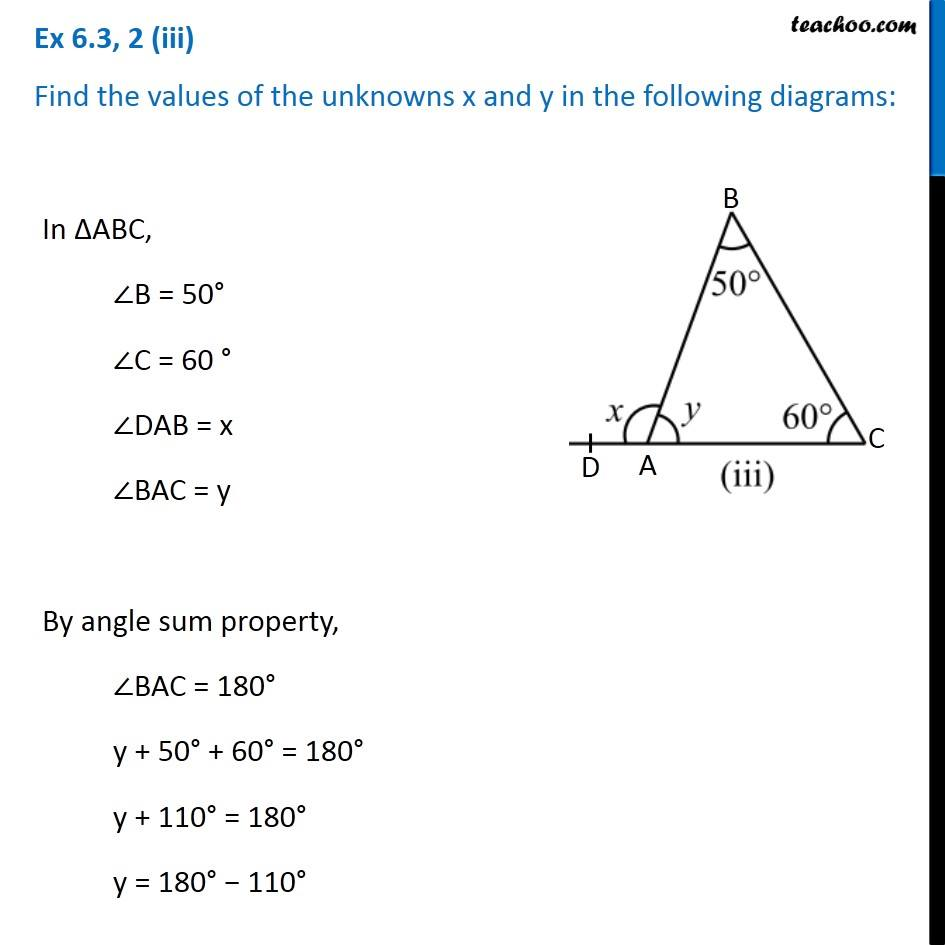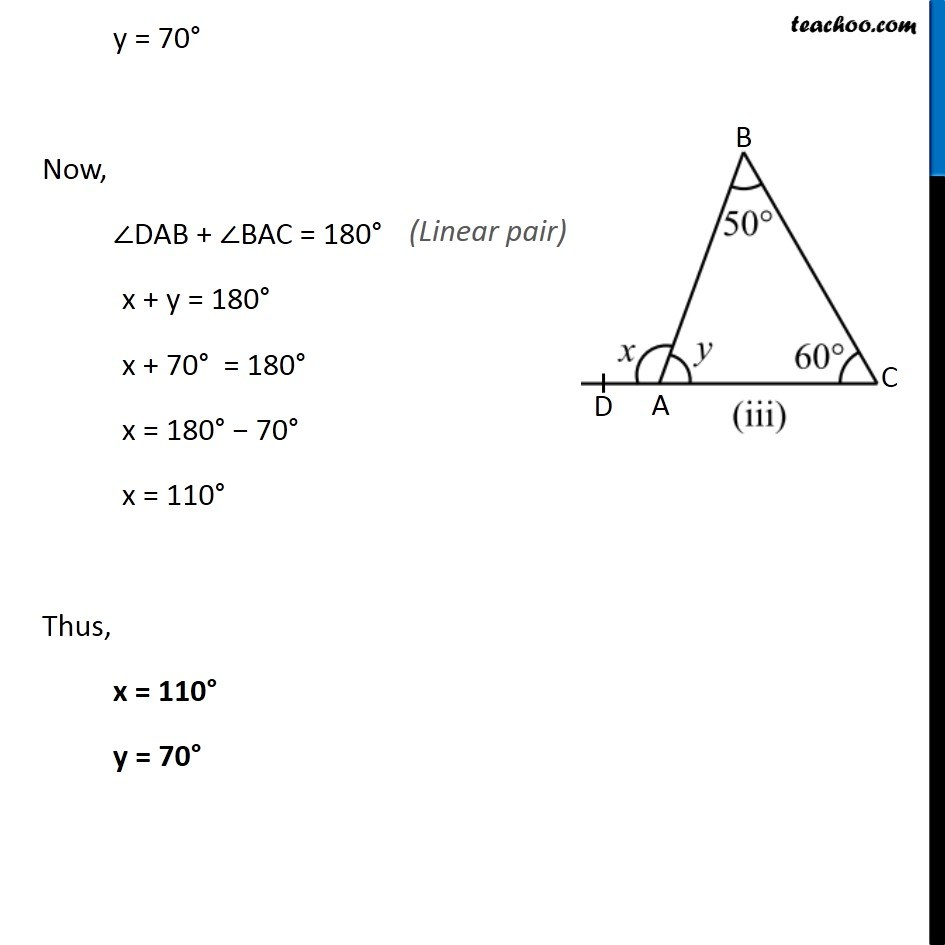Ex 6.3

Chapter 6 Class 7 Triangle and its Properties
Serial order wiseLearn in your speed, with individual attention - Teachoo Maths 1-on-1 Class

### Transcript

Ex 6.3, 2 (iii) Find the values of the unknowns x and y in the following diagrams:In ∆ABC, ∠B = 50° ∠C = 60 ° ∠DAB = x ∠BAC = y By angle sum property, ∠BAC = 180° y + 50° + 60° = 180° y + 110° = 180° y = 180° − 110° y = 70° Now, ∠DAB + ∠BAC = 180° x + y = 180° x + 70° = 180° x = 180° − 70° x = 110° Thus, x = 110° y = 70°